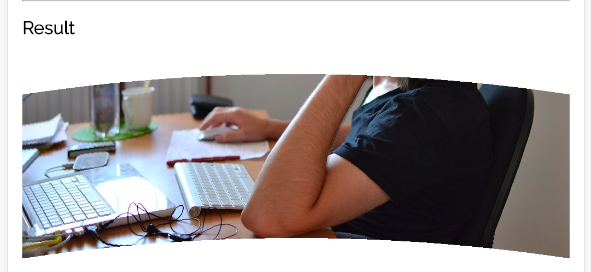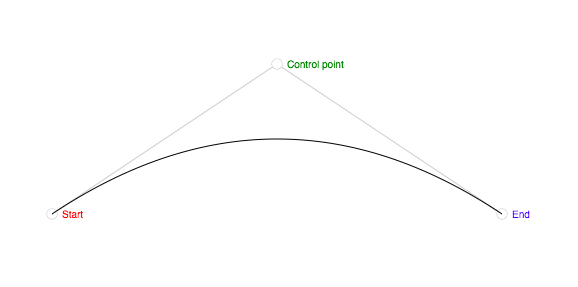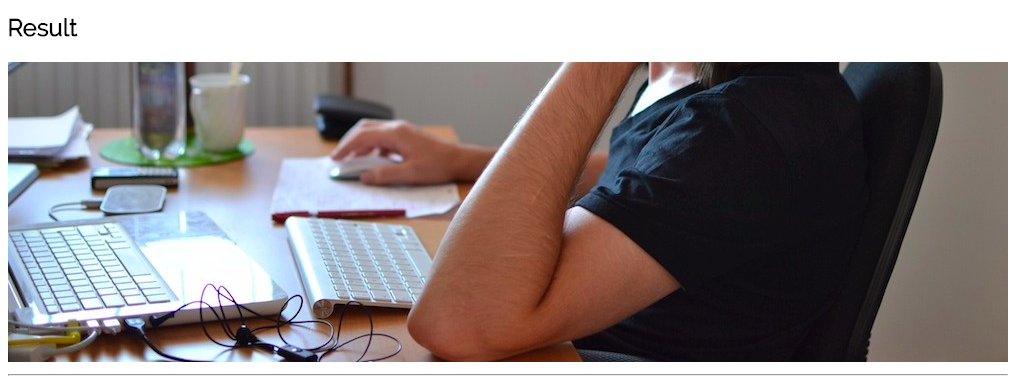Drawing a curved image with a HTML5 canvas and JavaScript

# Drawing a curved image with a HTML5 canvas and JavaScriptWhile building an online graphic editor based on FabricJS for one of our customers at Prestimedia, I ran into the need of showing a preview image curved (the kind that will go on a mug or a hat, for example). And while FabricJS is an amazing library that does pretty much all the heavy lifting, it lacks this particular option, finally leaving something for me to do.

Since FabricJS is canvas based all I had to do was find a general canvas solution and this StackOverflow question led me on the correct path: Fabric JS html 5 image curving options. The base idea is surprinsingly simple: what you have to do is draw the image pixel by pixel and applying a offset on the X or Y axis, according to your needs. Something like this:

``````var x = 0;
for ( ; x < image_width; x++ ) {
warp_context.drawImage(image_to_warp,
// clip 1 pixel wide slice from the image
x, 0, 1, image_height + warp_y_offset,
// draw that slice with a y-offset
x, warp_y_offset + offset_y_points[x], 1, image_height + warp_y_offset
);
}
``````

In this case, `warp_y_offset` is a pixel based value of the max offset, since the warped image will be automatically larger than the original one, due to the fact of being curved. `offset_y_points` is an array containing the offset value. For example, for the first pixel you draw the image with 0 offset, for the second and third pixel you draw it with an offset of 1 pixel, the fourth has 2px offset and so on.

In order to obtain a proper array of offsets according to the level of curving you desire, what you need is a quadratic curve. It might sound tricky and math-y but it's actually rather simple, as this StackOverflow answer points out: Drawing Shapes along a curved path in canvas

The main function is this one:

``````function getQuadraticBezierXYatT(start_point, control_point, end_point, T) {

var pow1minusTsquared = Math.pow(1 - T, 2),
powTsquared = Math.pow(T, 2);

var x = pow1minusT_squared * start_point.x + 2 * (1 - T) * T * control_point.x + powTsquared * end_point.x,
y = pow1minusT_squared * start_point.y + 2 * (1 - T) * T * control_point.y + powTsquared * end_point.y;

return {
x: x,
y: y
};
}
``````

and you use it like this to generate the array:

``````var offset_x_points = [],
t = 0;
for ( ; t < image_height; t++ ) {
var xyAtT = getQuadraticBezierXYatT(start_point, control_point, end_point, t / image_height),
x = parseInt(xyAtT.x);

offset_x_points.push(x);
}
``````

The start, control and end point might sound cryptic but they are actually quit simple. The best way of understanding them is visually and I found this example very helpful: An interactive example of the canvas quadraticCurveTo functionScreenshot taken from An interactive example of the canvas quadraticCurveTo function

How does it work when you piece everything? Well, you go from this...Does this look familiar to you?

to this:Curvy!

You can play with different ways of curving an image in this online demo: Image Curving Demo or check out the code on GitHub: Image Curving Demo